#Function Repository Resource:

# ANOVA

Perform an analysis of variance

Contributed by: Wolfram Research
 ResourceFunction["ANOVA"][data] performs a one‐way analysis of variance. ResourceFunction["ANOVA"][data,model,vars] performs an analysis of variance for model as a function of the categorical variables vars.

## Details and Options

The data can have the form {{x1,y1,,f1},{x2,y2,,f2},}, where the number of coordinates x,y, is equal to the number of variables in the list vars.
For data of the form {{x1,f1},{x2,f2},}, a one‐way analysis of variance can be obtained without explicitly specifying the model and variable.
The model argument can be a list containing main effects and interactions. Main effects are elements of vars, and interactions are products of main effects.
A full factorial model including all interactions between variables x,y, can be specified as {x,y,,All}.
The following options can be given:
 "CellMeans" True whether cell means should be included in the output WorkingPrecision MachinePrecision the precision used in internal computations "PostTests" { } significance tests to perform SignificanceLevel 0.05 significance level for performed tests
Possible settings for "PostTests" include: "Bonferroni", "Duncan", "Dunnett", "StudentNewmanKeuls", and “Tukey”.

## Examples

### Basic Examples

A one‐way analysis of variance:

 In:=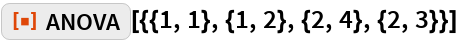Out=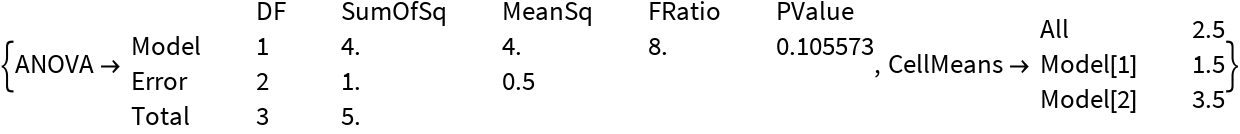A two‐way analysis of variance:

 In:=Out=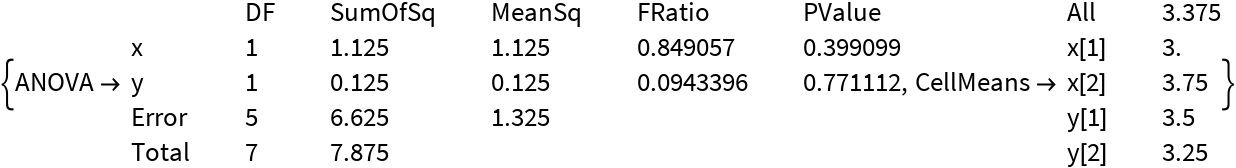### Options

#### CellMeans

Omit cell means from output:

 In:=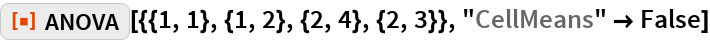Out=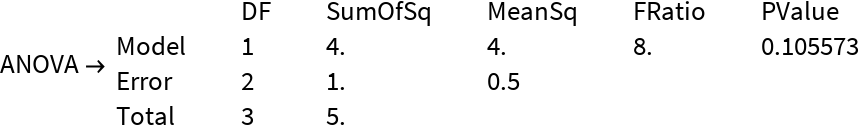#### WorkingPrecision

Computation at precision 20:

 In:=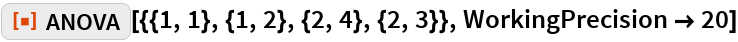Out=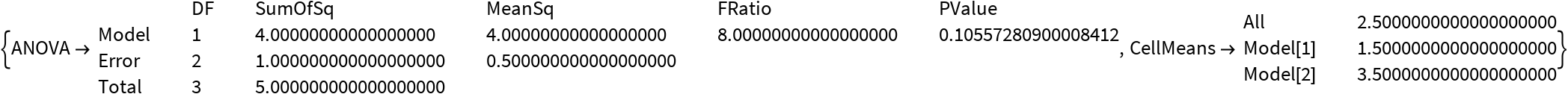#### PostTests

Analysis of variance with Bonferroni test:

 In:=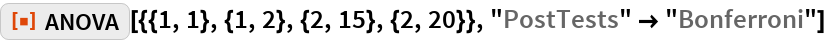Out=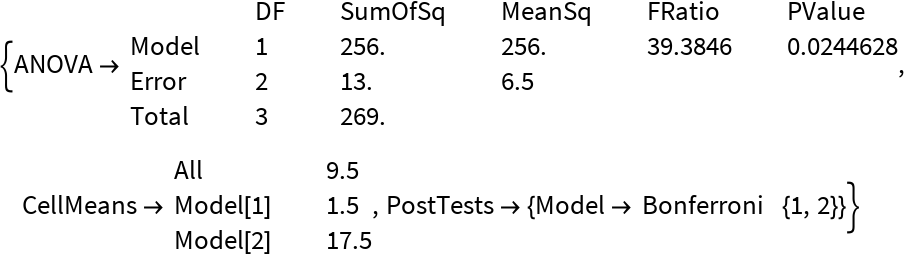#### SignificanceLevel

Bonferroni test at significance level .01:

 In:=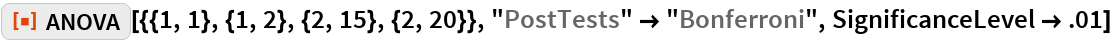Out=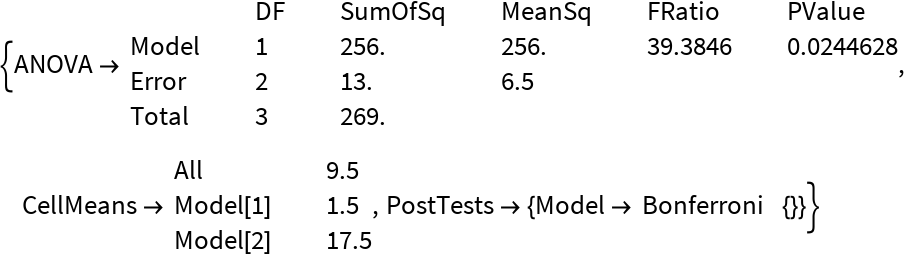## Requirements

Wolfram Language 11.3 (March 2018) or above